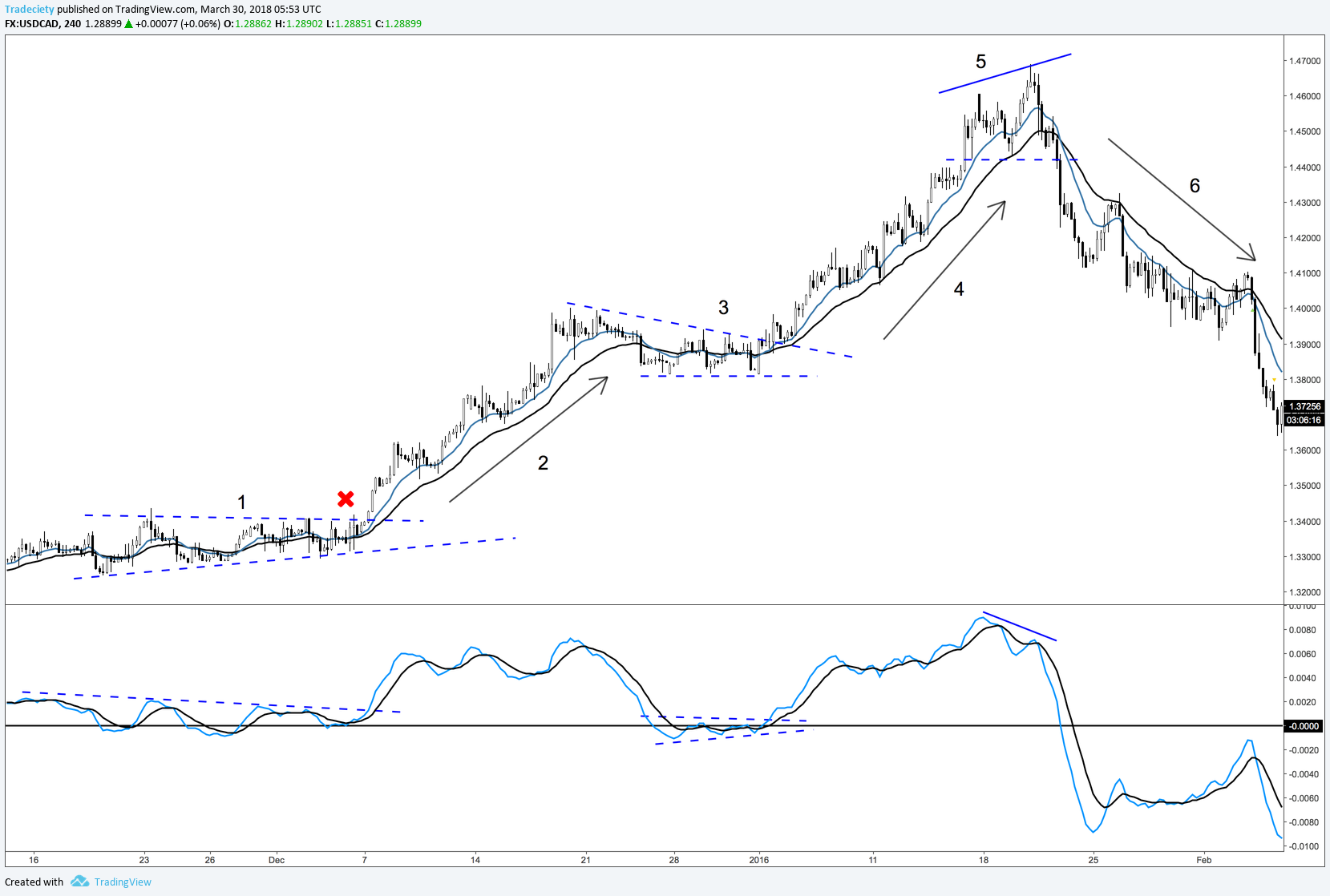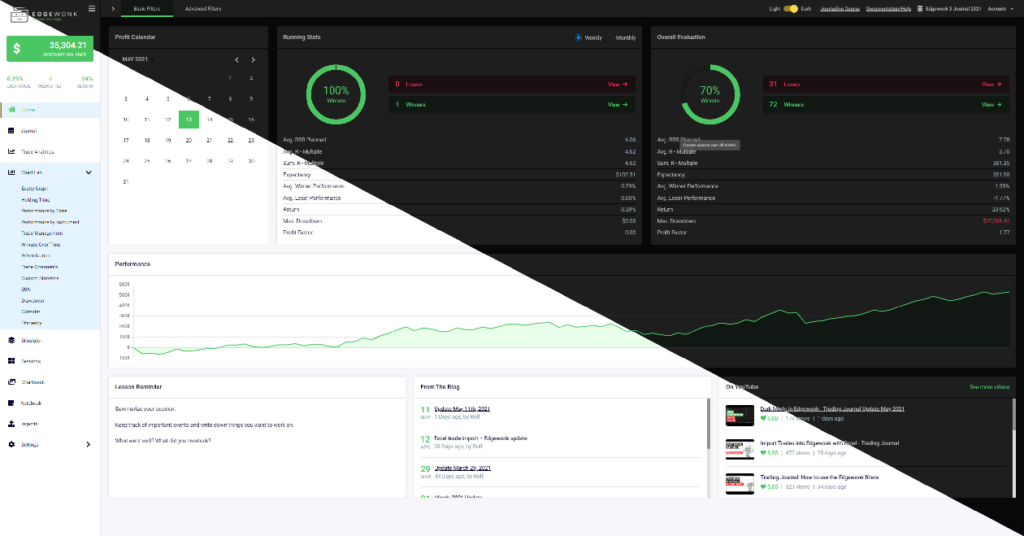The Fibonacci tool is very popular amongst traders and for good reasons. The Fibonacci is a universal trading concept that can be applied to all timeframes and markets. There are also countless Fibonacci tools from spirals, retracements, Fib time zones, Fib speed resistance to extension.

In this article, I will explain how to correctly draw a Fibonacci sequence and how to use the Fibonacci extensions for your trading.

### How to draw Fibonaccis – just do it

Often, traders who have no prior experience with Fibonaccis are worried that they are ‘doing it wrong’ and they then don’t use the Fibonacci tool at all. I can assure you, there is no right or wrong when it comes to drawing Fibonacci and you will also see that different traders use Fibonacci in slightly different ways.

The Fibonacci levels are %-based which means that even when you draw them differently, they will often line up correctly.

## Step 1 –  Find an ‘A to B’ move

To use the Fibonacci retracements, you have to first identify an ‘A to B’ move where you can use the Fibonacci retracement tool. What do we mean with ‘A to B’?

A = the origin of a new price or trend move. These are usually swing highs and lows, or tops and bottoms.

B = Where the trend move pauses and reverses to make a retracement.

### The following 4 screenshots show typical A to B moves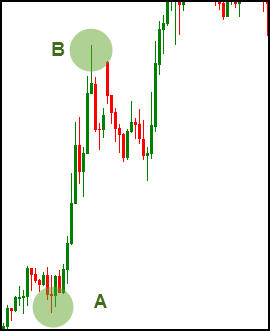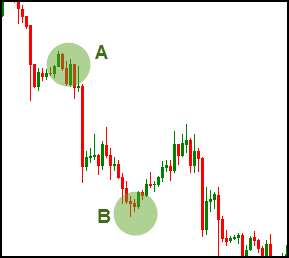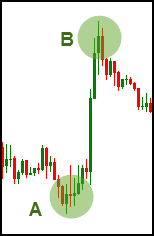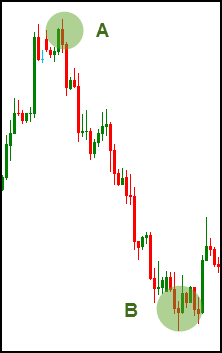Now let’s apply the Fibonacci retracement tool to the A to B moves. For that, we pick the Fibonacci tool from your platform, select point ‘A’, drag it to ‘B’ and release it.

### Connecting A to B moves with the Fibonacci retracement tool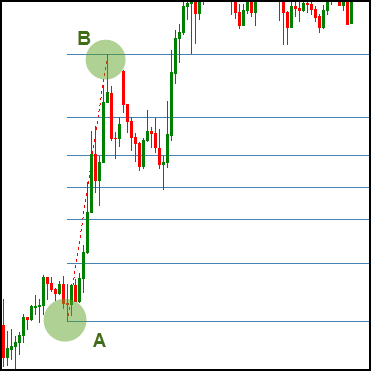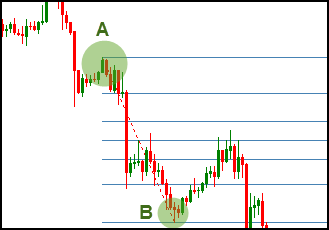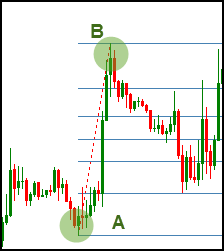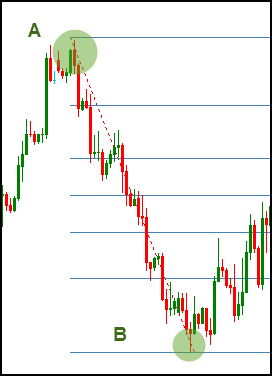## Step 2 – Find the retracement point C

After you have identified an A to B move and plotted your Fibonacci tool on your charts, you should be able to find point C.

C = the point where the retracement ends and price reverses into the original direction.

As you can see, the first 3 screenshots show the typical ABC move of a Fibonacci retracement. Point C is very obvious on all three charts and price bounced off the Fibonacci levels accurately.

### Finding the C-Fibonacci retracement level

The fourth screenshot shows a scenario where price did not go back to the B-Fibonacci level, but breaks the prior A-Fibonacci. It’s important to understand that not all price moves will stop at a Fibonacci level. But, as you can see on the fourth screenshot, the Fibonacci tool can be used to identify support and resistance areas as well as we will explore in more detail shortly; the last screenshot shows nicely how price reacts to several different Fibonacci levels during the retracement.

### Tip #1: Trial and error

Especially for beginners, the following exercise will help you build a strong foundation when it comes to drawing Fibonacci levels: Just grab the Fibonacci retracement tool and try to put it on different spots, while observing how price reacts to it. Usually, the more ‘snaps’ (price bouncing off a level) you see, the more important the Fibonacci retracement is.

### Tip #2: Don’t force a Fibonacci

Not every time you’ll be able to use a Fibonacci retracement to make sense of a price move. If you can’t make the Fibonacci levels snap, don’t try to force it. The best and most helpful Fibonacci retracements are those where you don’t have to look long.

## How to trade with Fibonacci

### #1 Retracements as re-entries

The most common use for Fibonacci levels is the regular retracement strategy. After identifying the ‘A to B’ move, you pay attention to the retracement level C.

The screenshots below show a sudden bullish move in a larger uptrend. Often, traders miss such sudden outbursts and then try to find re-entries during pullbacks. The Fibonacci tool is ideal to identify swing-points during pullbacks as the sequence indicates. With the Fibonacci retracement tool, a trader would have been able to find 2 Fibonacci re-entries on the pullbacks.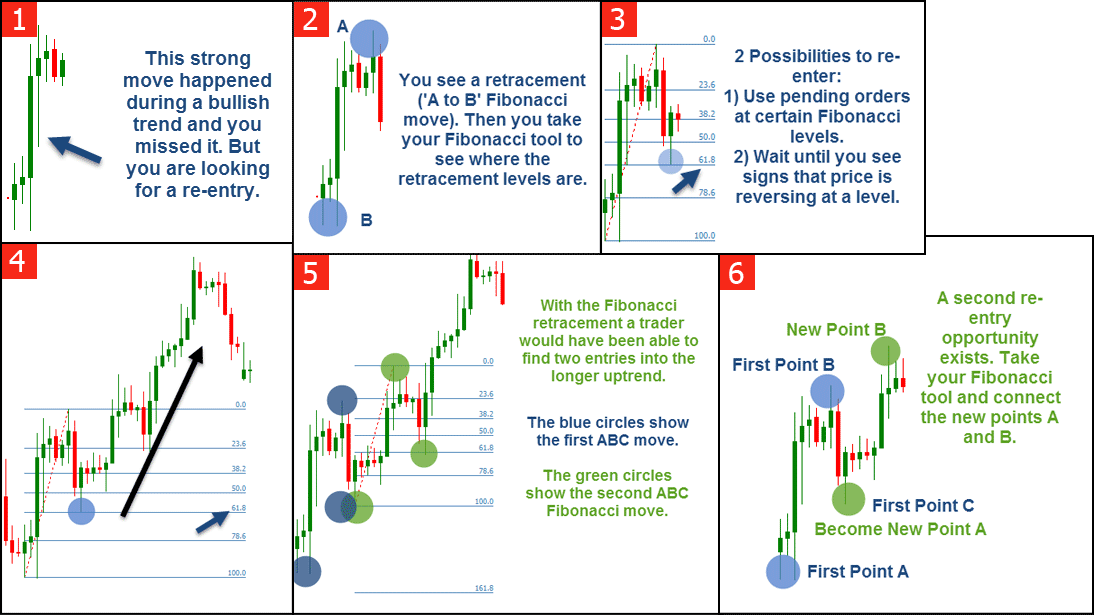[/sociallocker] Using Fibonacci retracements as re-entries in a trade – click to enlarge

### #2 Support and resistance

Another possibility to use Fibonaccis is to find an AB-Fibonacci move on a higher timeframe and then go down to your regular timeframe and watch the retracement levels as support and resistance guidelines.

The first screenshot below shows the Daily timeframe of the current EUR/USD chart. As you can see, there was a regular ‘A to B’ move. The screenshot in the bottom shows the same Fibonacci retracement but on the lower, 4 hour timeframe. As you can see, throughout the whole time, price reacted fairly accurately to the Fibonacci levels.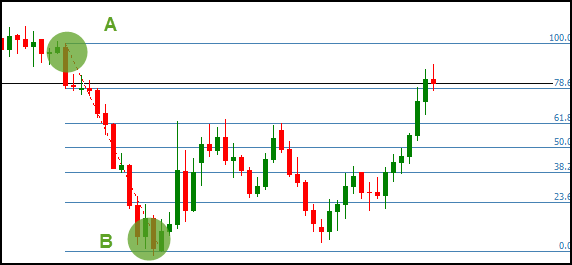Daily timeframe with an ‘A to B’ Fibonacci move – click to enlarge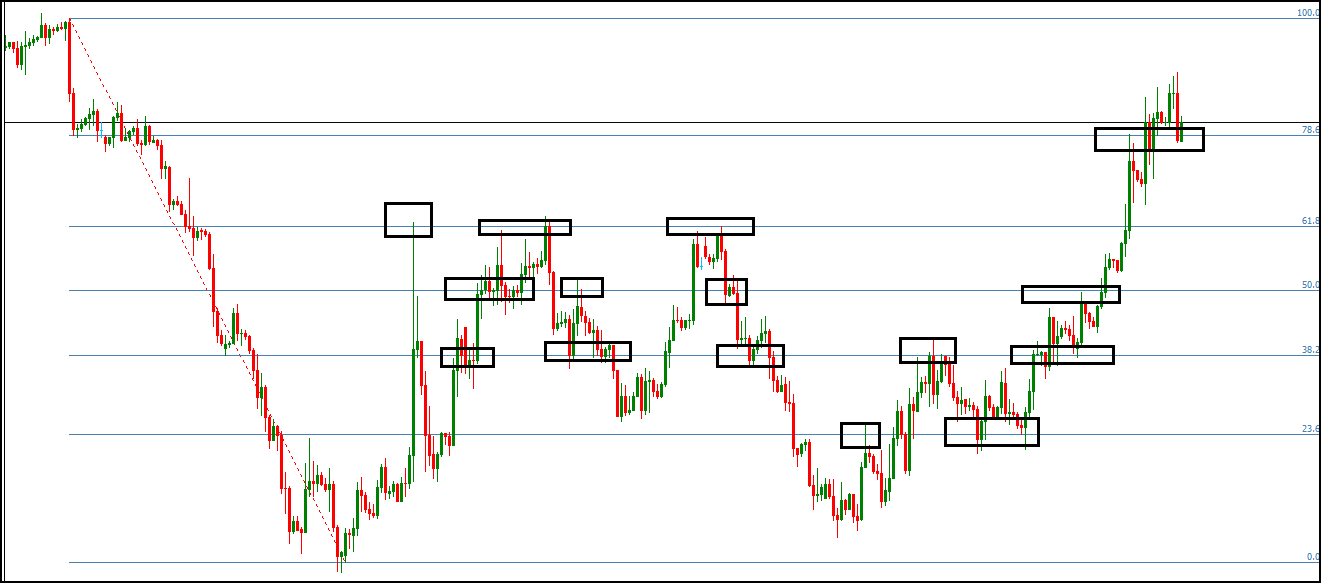Fibonacci levels acting as support and resistance on a lower timeframe – click to enlarge

### #3 Fibonacci levels for Take Profits – Fibonacci Extensions

Finally, you can also use Fibonaccis for your take profit orders. Especially the Fibonacci extensions are ideal to determine take profit levels in a trend. The most commonly used Fibonacci extension levels are 138.2 and 161.8.

Most trading platforms allow you to add custom levels. Usually, the parameters to add the Fibonacci extensions are:

-0.618 for the 161.8 Fibonacci extension

-0.382 for the 138.2 Fibonacci extension

The rules for take profit orders are very individual, but most traders use it as follows:

A 50, 61.8 or 78.6 retracement will often go to the 161 Fibonacci extension after breaking through the 0%-level. A 38.2 retracement will often come to a halt at the 138 Fibonacci extension. The screenshots below show the Fibonacci moves from the beginning and this time we applied the extensions to the price moves. As you can see, the extensions provided great places for take profit orders.

In my strategy, I use the Fibonacci extensions to find trends that have completed an ABCD pattern and are likely to reverse. The Fib extension can be of great help here.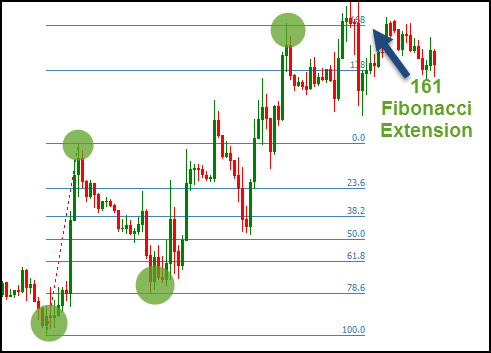A 78.6 retracement goes to the 161 Fibonacci extension – click to enlarge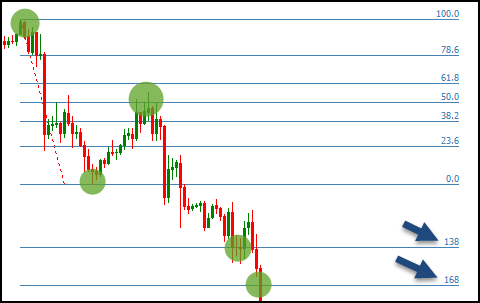A 50 retracement goes to the 168 Fibonacci retracement – click to enlarge

## Conclusion: Fibonaccis are multifunctional

The article demonstrated how to use Fibonaccis efficiently in your trading. However, don’t make the mistake of idealizing FIbonaccis and believing that they are superior over other tools and methods. Nevertheless, Fibonacci is a great tool to have and can be used very effectively as another confirmation method. Whether you are a trend following or a support and resistance trader, or just looking for ideas how to place your take profit orders, Fibonaccis are a great addition to your arsenal.I set out with the goal to create the trading program that...